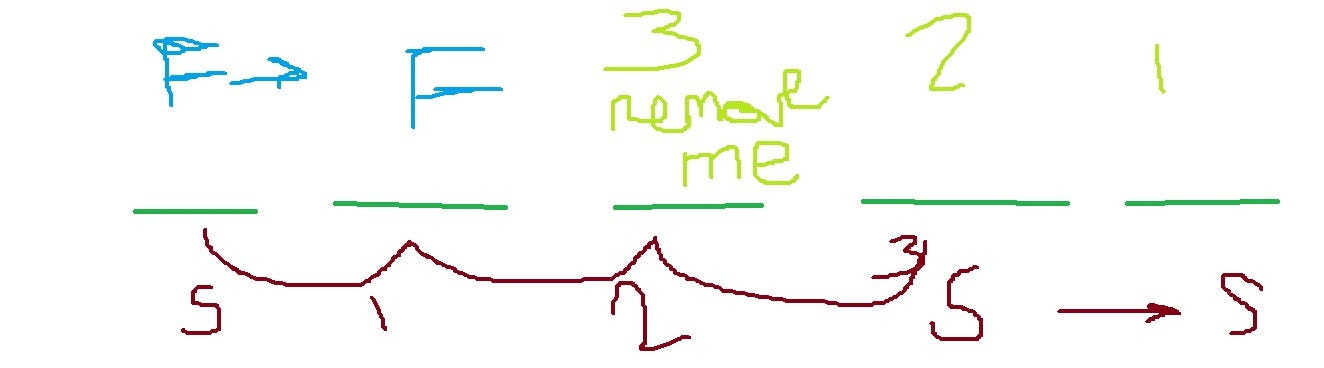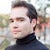# CoDEVIANT #25 — Linked List Surgery

• Takes an integer k
• Removes the kth node from the end of the Linked List
• If the head of the linked list turns out to be the one we’re supposed to remove, we simply update its value and next-pointer.
• the byte(s) of memory that holds the node’s value, and
• the byte(s) of memory pointing to the address of the chunk that is the start of the next node.
`let counter = 1class LinkedList {  constructor(value) {    this.value = value;    this.next = null;  }}function removeKthNodeFromEnd(head, k) {  counter = 1  processNode(head, 1)  let calculatedNumber = counter - k  if(calculatedNumber != 0) {    clipTreeLinks(head, calculatedNumber) }  else {  head.value = head.next.value  head.next = head.next.next }}function processNode(node, number) { if(node.next != null) {  counter = number + 1  processNode(node.next, counter) } }function clipTreeLinks(node, number) { let clipCounter = 0while(clipCounter < number ) {  if(node.next && node.next != null) {   if(clipCounter == number - 1 ) {    node.next = node.next.next   }  clipCounter++  }  node = node.next }}`
`let counter = 1class LinkedList {  constructor(value) {    this.value = value;    this.next = null;  }}`
`let counter = 1`
`function removeKthNodeFromEnd(head, k) {  counter = 1  processNode(head, 1)  let calculatedNumber = counter - k  if(calculatedNumber != 0) {    clipTreeLinks(head, calculatedNumber) }  else {  head.value = head.next.value  head.next = head.next.next }}`
• We set counter, our global variable to 1 (in case it happens to not be 1 at the moment)
• We call the method processNode with the arguments of head and 1. It should be mentioned at this time that the code of processNode will impact what ‘counter’ is.
• Then we declare a variable called calculatedNumber that will be the difference of what counter is at that point and k.
• If calculatedNumber is not zero, which also means that we would not be trying to remove the head of the linked-list, then we call clipTreeLinks and pass in the head and the calculatedNumber.
• If calculatedNumber IS zero, however, we make the head’s value equal the following node’s value
• Also, if calculatedNumber IS zero, we make the head’s next value equal the following node’s next value.
`function processNode(node, number) { if(node.next != null) {  counter = number + 1  processNode(node.next, counter) } }`
• First, we look at the passed in node’s next value. If it is NOT null, then we make the global variable counter equal the passed in number plus 1. Afterwards, we call processNode on the current node’s next value and pass in our newly updated counter as the number-argument.
• So this will go on over and over until we reach the tail of the linked-list. The tail is the node in the linked list at the end where the next property is null, at which point, we enter the implicit else portion of the if-else statement where nothing happens.
`let calculatedNumber = counter - k`
`if(calculatedNumber != 0) {    clipTreeLinks(head, calculatedNumber) }  else {  head.value = head.next.value  head.next = head.next.next }`
`function clipTreeLinks(node, number) { let clipCounter = 0while(clipCounter < number ) {  if(node.next && node.next != null) {   if(clipCounter == number - 1 ) {    node.next = node.next.next   }  clipCounter++  }  node = node.next }}`
• First we say that clipCounter is zero.
• We say that while clipCounter is less than the number argument that was passed in, if node.next exists, then in MOST cases, we will simply increment clipCounter by one and say the node is now the following node.
• However if clipCounter equals the number minus 1….THEN we say that node.next will be the subsequent node’s next
`if(clipCounter == number - 1 ) {    node.next = node.next.next}`
`function removeKthNodeFromEnd(head, k) {  let counter = 1 let first = head let second = head  while(counter <= k) {  second = second.next  counter += 1 } if second == null {  //this means the value we wanted to remove from a 10 lenth list is the 10th, which means the frist pointer (head)  //is at the node that needs updating   head.value = head.next.value   head.next = head.next.next  return } while(second.next != null) {  second = second.next  first = first.next } //once the second goes fast enough to get to null,  //then we know that first.next needs to be first.next.next first.next = first.next.next}`
`while(counter <= k) {  second = second.next  counter += 1 }`
`if second == null {   head.value = head.next.value   head.next = head.next.next  return }`
`first.next = first.next.next`Here’s a crude image of k being 3. Where we send ‘second’ out 3 spaces. and then once more so that second.next equals null. At which point first.next needs to become first.next.next

--

--

--

is a web developer, opera singer, actor, and lover of cats. (adrian-rosales.tech)

Love podcasts or audiobooks? Learn on the go with our new app.

## How to speed up a Tree structure traversal in Go?## Strava killed Relive’s API Access## Windows 11 is great, and you can’t tell me otherwise## 10 SQL Queries You Should Know as a Data Scientist## TryHackMe:Gaming Server[CTF]## D3.js — What’s new in Version 6?## Automate Coverage Reports in Pull Requests with Bitbucket, Jenkins and SonarCloud — Bitbucketis a web developer, opera singer, actor, and lover of cats. (adrian-rosales.tech)

## How to Style for the Transitioning Seasons## Let’s Try This Again…## OVERVIEW OF KNIGHT ECOSYSTEM Everybody likes to have a Wizard, Dragon & or Knight guarding their…